### MelAlign Y Axis to Vector

#### Introduction

Occasionally it is necessary to apply a series of rotations to a coordinate system so that one of its axes points in a specific direction. This tutorial explains how the y-axis of a coordinate system can be aligned to a direction specified by a vector.

Although the calculations shown on this page were derived from "The RenderMan Companion" by Steve Upstill (pages 142/143) I have altered them slightly because RenderMan uses a left-hand coordinate system whereas Maya uses a right hand system. An additional difference between Upstill's calculations and mine is that his code transforms the (RenderMan) camera coordinate system.

Despite these differences the method by which a coordinate system is transformed is essentially the same in both Upstill's "AimZ" function and my "AimY" procedure. If you have studied Upstills code and have wondered why his calculations work I hope the illustrations on this page makes the mathematics he uses a little clearer.

#### Coordinate Axes

Fig 1 shows a vector with coordinates [-2,2,1]. The pale red trace lines show the vector projected onto the x-y plane, the x-z plane and the y-z plane. The easiest way of figuring out how to rotate the coordinate system so that the y-axis points in the direction of the vector is to think about the problem in reverse! How can the vector be aligned to the current y-axis. The vector in question is shown below in black - coordinates [-2, 2, 1].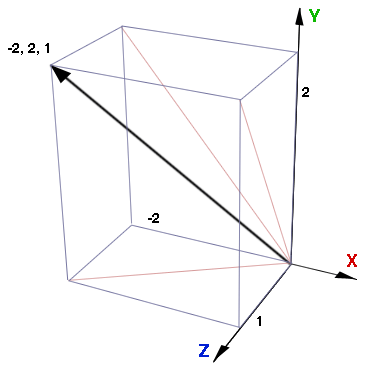Figure 1

#### Rotations

##### Step 1

Figure 2 shows the vector forms the hypotenuse a right angled triangle.

##### Step 2

Figure 3 shows the triangle can be aligned to the y-z plane by applying a suitable rotation around the z-axis.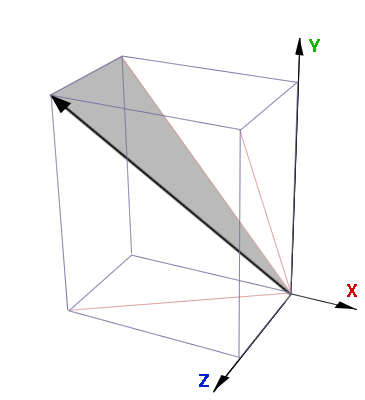Figure 2 - vector forms a triangle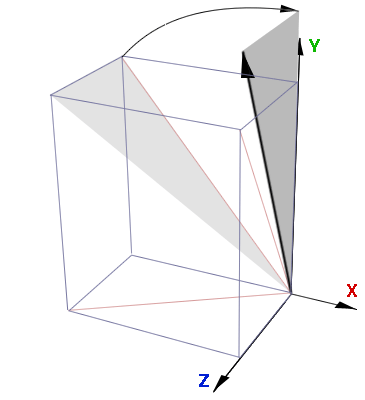Figure 3 - rotation around the z-axis

##### Step 3

Fig 3 shows that a rotation around the x-axis will align the vector to the y-axis of the coordinate system (fig 4).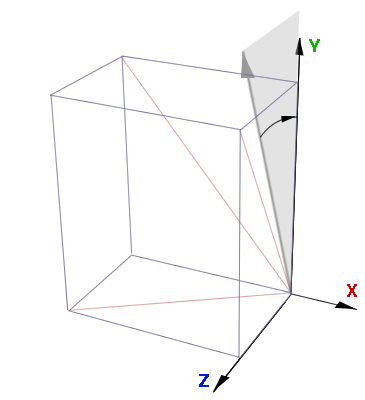Figure 4 - rotation around the x-axis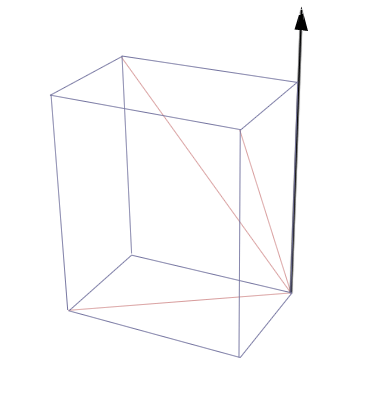Figure 5 - vector aligned with the y-axis

#### Angles

Figure 6 shows two angles that must be calculated in order to perform steps 2 and 3. To find the blue angle we must first calculate the length of the red trace line, xyLength, on the x-y plane ie.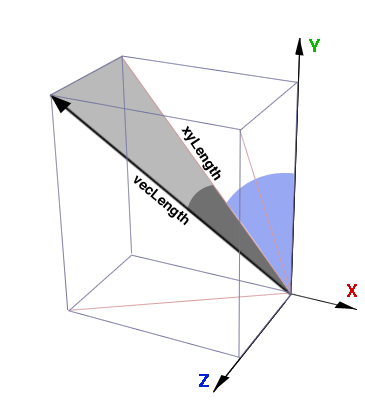Figure 6 - the two principle angles

```    xyLength = sqrt(x * x + y * y);
xyLength = sqrt(-2 * -2 + 2 * 2);
xyLength = 2.83;
```

After which the angle shown in blue can be found,

```    zAngle = acos(y / xyLength);
zAngle = acos(2.0 / 2.83);
zAngle = 0.785;	/* acos returns angle in radians */
```

To find the dark gray angle the length of the vector must be found,

```    vecLength = sqrt(x * x + y * y + z * z);
vecLength = sqrt(-2 * -2 + 2 * 2 + 1 * 1);
vecLength = 3.0;
```

As with the blue angle the angle in dark gray is found from the cosine. I am referring to this angle as the xAngle because, as shown in Fig 4, this angle will be used to define the rotation around the x-axis.

```    xAngle = acos(xyLength / vecLength);
xAngle = acos(2.83 / 3.0);
xAngle = 0.338;
```

Expressed in degrees the zAngle is 45.0 and the xAngle is 19.4. Therefore, the rotations needed to orientate the y-axis in the direction of the vector requires,

1. a rotation around the x-axis of 19.4 degrees, then,
2. a rotation around the z-axis of -45 degrees

A mel procedure that implements this technique is given in listing 1.

Listing 1

 ```proc float[] aimY(vector \$vec) { float \$out; float \$xAngle, \$zAngle, \$xyLength, \$vecLength; \$xyLength = sqrt((\$vec.x) * (\$vec.x) + (\$vec.y) * (\$vec.y)); \$vecLength = sqrt((\$vec.x) * (\$vec.x) + (\$vec.y) * (\$vec.y) + (\$vec.z) * (\$vec.z)); // \$xyLength will be zero when \$vec is pointing // along the +z or -z axis if(\$xyLength == 0) \$zAngle = (\$vec.x) > 0 ? deg_to_rad(90) : deg_to_rad(-90); else \$zAngle = acos((\$vec.y)/\$xyLength); \$xAngle = acos(\$xyLength/\$vecLength); \$xAngle = (\$vec.z) > 0 ? \$xAngle : -\$xAngle; \$out = rad_to_deg(\$xAngle); \$zAngle = (\$vec.x) > 0 ? -\$zAngle : \$zAngle; \$out = rad_to_deg(\$zAngle); return \$out; } ```

#### Checking the Script

The following script uses the aimY() procedure to point a cone along a vector. Fig 7 shows that the apex of the cone does extend along the vector - the coordinates of which are indicated by the gray box.Figure 7 - a cone aligned to a direction vector

```    vector \$v = <<-2,2,1>>;
float \$a[] = aimY(\$v);

// begin with an empty scene
select -all;
delete;

// insert a cone aligned to the y-axis
cone -r 0.2 -hr 17 -ax 0 1 0;

// apply the rotations to the x
// and z axes of the cone
rotate -r \$a 0  0;
rotate -r 0     0  \$a;

// draw a box to indicate the coordinates of the vector
polyCube -w  2 -h 2 -d 1;
move -r -1 1 0.5;```